• ") print('=' * 70) # 检测异常值 outlier = data[(data[column] ) | (data[column] >= upper)] return outlier, upper, lower 调用函数 outlier, upper, lower = outlier_test(data=df, column='price', method='z'...

1、、数据清洗

1.1、数据缺失，即存在某些数据等于0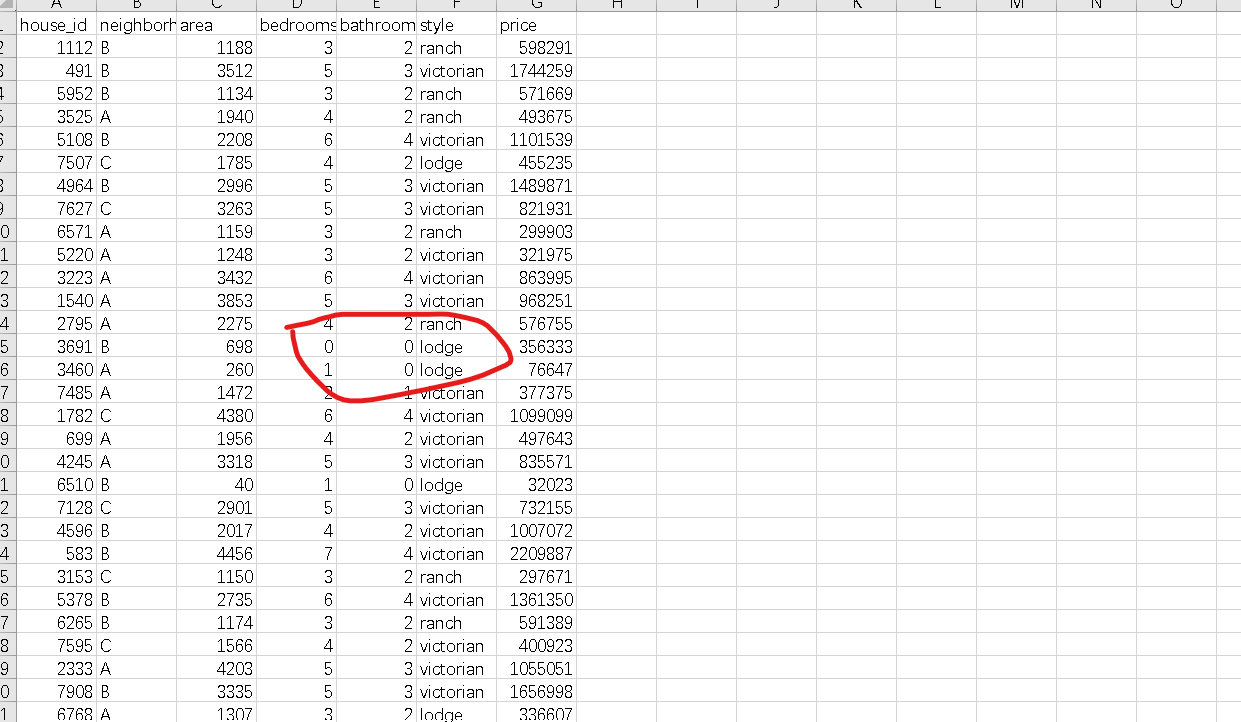解决办法：选中缺失数据的列，然后采用选择菜单：点击数据——筛选，选中数据是0的，点击确定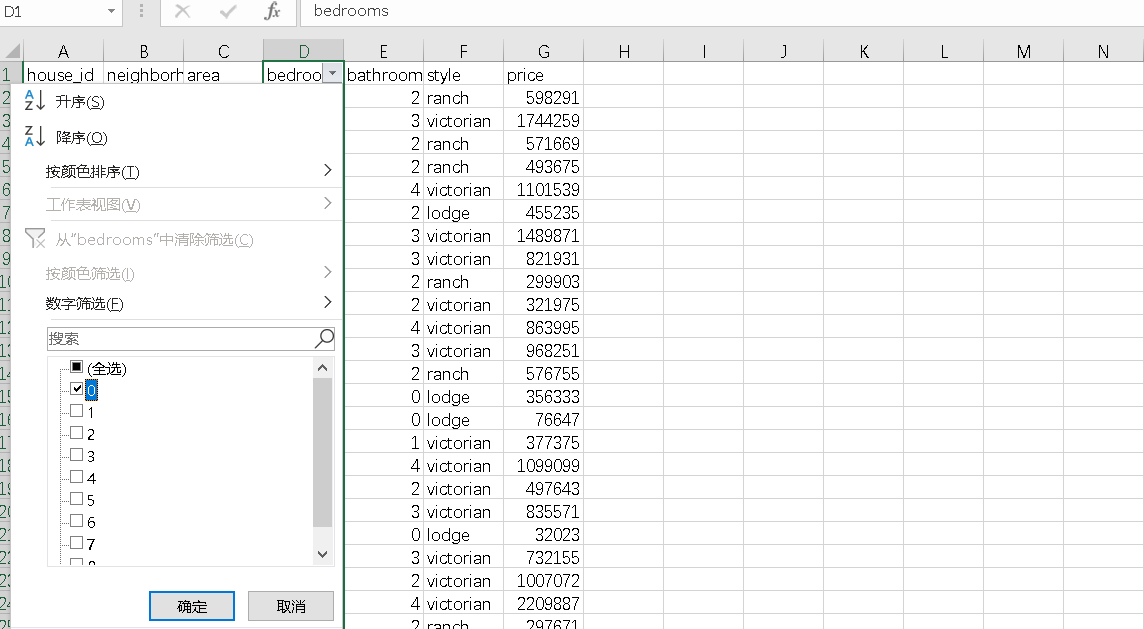然后点击删除行即可以删除数据同样的操作删除后一列bathroom的缺失值。

1.2、存在重复数据

解决办法：excel打开数据集，选中需要处理的数据，然后选择数据——数据工具——删除重复值，在弹出的窗口里利用唯一标识house_id，删除重复值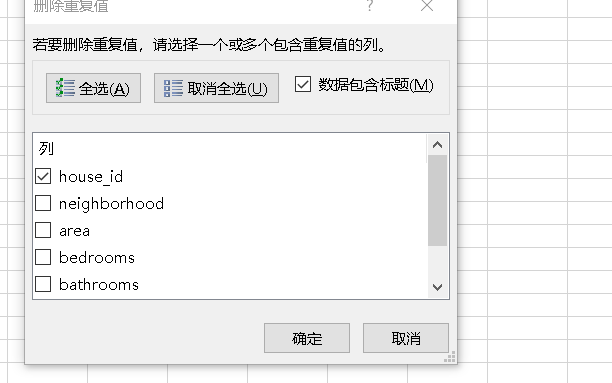1.3、存在非数值性属性

原始数据中的neighborhood和style为非数值型数据，需要转换成数值型数据才能够进行回归分析。
解决办法：选中开始——查找和替换——替换全部替换完成所有A的转换，同理进行B和C以及style的替换
完成清理之后的数据对数据进行保存

2、多元线性回归代码实现

2.1、基础包、数据导入

import pandas as pd
import numpy as np
import seaborn as sns
from sklearn import datasets
from sklearn.linear_model import LinearRegression
df.info()#显示列名和数据类型类型

导入包并读取导入包读取文件house_prices.csv’数据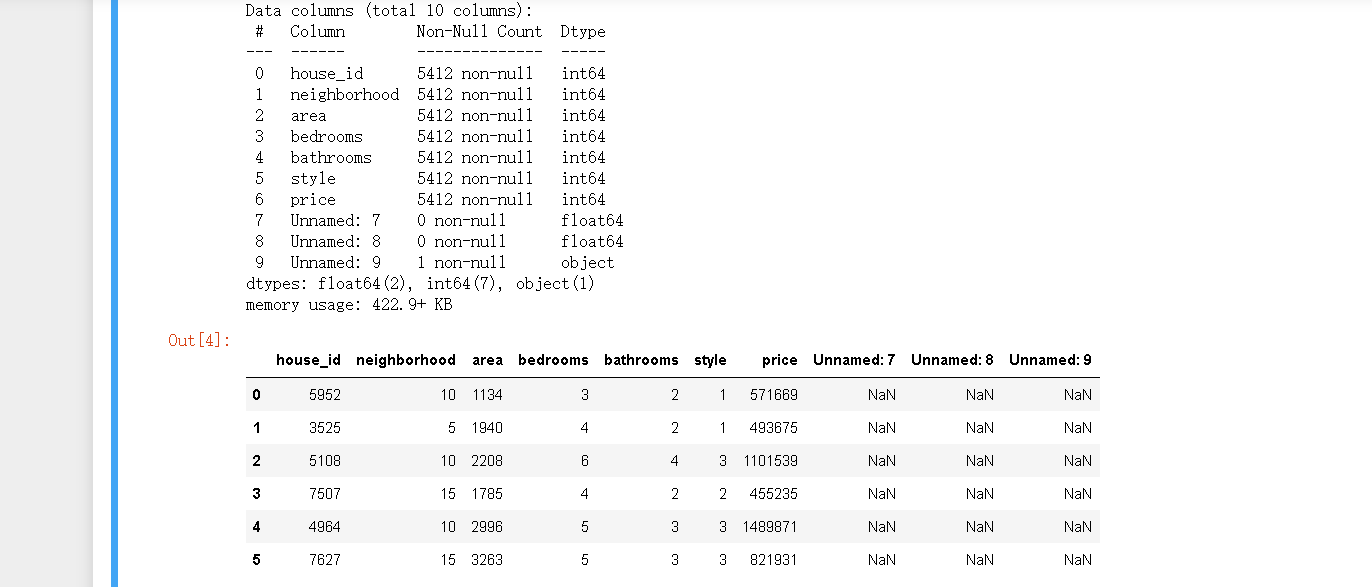2.2、数据处理、探索

进行数据处理

# 异常值处理
# ================ 异常值检验函数：iqr & z分数 两种方法 =========================
def outlier_test(data, column, method=None, z=2):
""" 以某列为依据，使用 上下截断点法 检测异常值(索引) """
"""
full_data: 完整数据
column: full_data 中的指定行，格式 'x' 带引号
return 可选; outlier: 异常值数据框
upper: 上截断点;  lower: 下截断点
method：检验异常值的方法（可选, 默认的 None 为上下截断点法），
选 Z 方法时，Z 默认为 2
"""
# ================== 上下截断点法检验异常值 ==============================
if method == None:
print(f'以 {column} 列为依据，使用 上下截断点法(iqr) 检测异常值...')
print('=' * 70)
# 四分位点；这里调用函数会存在异常
column_iqr = np.quantile(data[column], 0.75) - np.quantile(data[column], 0.25)
# 1，3 分位数
(q1, q3) = np.quantile(data[column], 0.25), np.quantile(data[column], 0.75)
# 计算上下截断点
upper, lower = (q3 + 1.5 * column_iqr), (q1 - 1.5 * column_iqr)
# 检测异常值
outlier = data[(data[column] <= lower) | (data[column] >= upper)]
print(f'第一分位数: {q1}, 第三分位数：{q3}, 四分位极差：{column_iqr}')
print(f"上截断点：{upper}, 下截断点：{lower}")
return outlier, upper, lower
# ===================== Z 分数检验异常值 ==========================
if method == 'z':
""" 以某列为依据，传入数据与希望分段的 z 分数点，返回异常值索引与所在数据框 """
"""
params
data: 完整数据
column: 指定的检测列
z: Z分位数, 默认为2，根据 z分数-正态曲线表，可知取左右两端的 2%，
根据您 z 分数的正负设置。也可以任意更改，知道任意顶端百分比的数据集合
"""
print(f'以 {column} 列为依据，使用 Z 分数法，z 分位数取 {z} 来检测异常值...')
print('=' * 70)
# 计算两个 Z 分数的数值点
mean, std = np.mean(data[column]), np.std(data[column])
upper, lower = (mean + z * std), (mean - z * std)
print(f"取 {z} 个 Z分数：大于 {upper} 或小于 {lower} 的即可被视为异常值。")
print('=' * 70)
# 检测异常值
outlier = data[(data[column] <= lower) | (data[column] >= upper)]
return outlier, upper, lower

调用函数

outlier, upper, lower = outlier_test(data=df, column='price', method='z')
outlier.info(); outlier.sample(5)

删除错误数据

# 这里简单的丢弃即可
df.drop(index=outlier.index, inplace=True)

定义变量进行数据分析

# 类别变量，又称为名义变量，nominal variables
nominal_vars = ['neighborhood', 'style']

for each in nominal_vars:
print(each, ':')
print(df[each].agg(['value_counts']).T)
# 直接 .value_counts().T 无法实现下面的效果
## 必须得 agg，而且里面的中括号 [] 也不能少
print('='*35)
# 发现各类别的数量也都还可以，为下面的方差分析做准备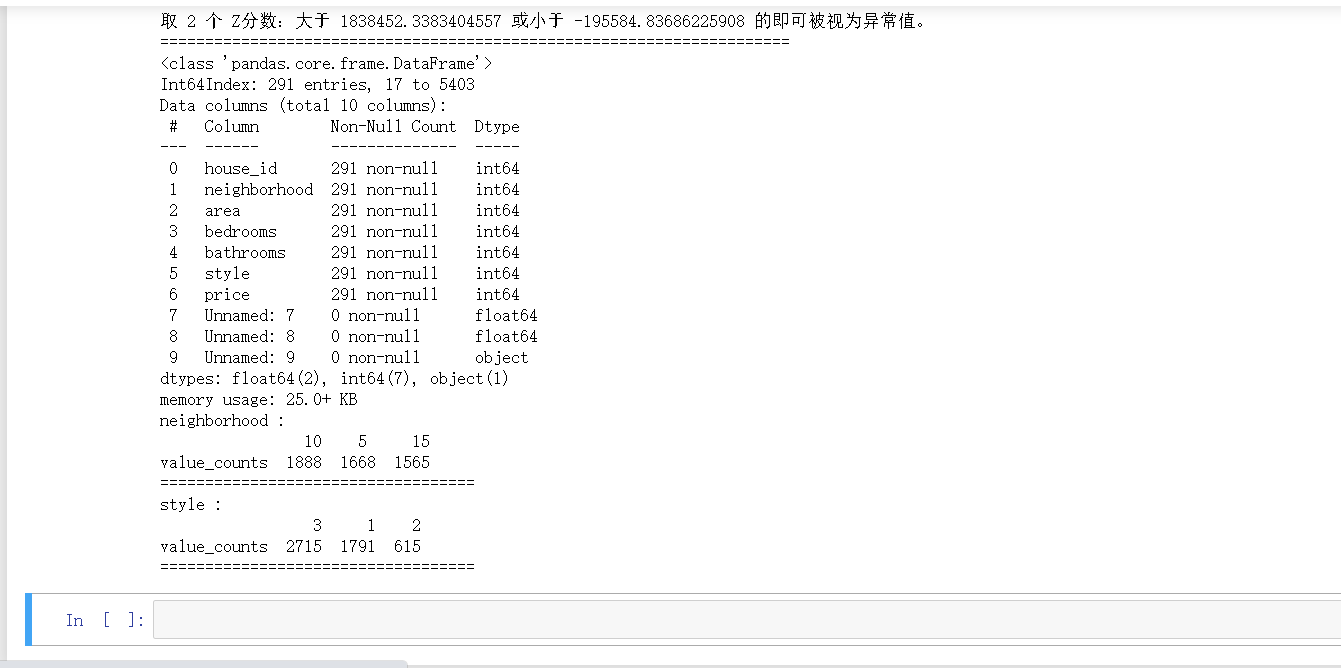调用热力图查看各变量之间的关联性

# 热力图
def heatmap(data, method='pearson', camp='RdYlGn', figsize=(10 ,8)):
"""
data: 整份数据
method：默认为 pearson 系数
camp：默认为：RdYlGn-红黄蓝；YlGnBu-黄绿蓝；Blues/Greens 也是不错的选择
figsize: 默认为 10，8
"""
## 消除斜对角颜色重复的色块
plt.figure(figsize=figsize, dpi= 80)
sns.heatmap(data.corr(method=method), \
xticklabels=data.corr(method=method).columns, \
yticklabels=data.corr(method=method).columns, cmap=camp, \
center=0, annot=True)

然后调用函数输出结果

heatmap(data=df, figsize=(6,5))

查看其热力图， 通过热力图可以看出 area，bedrooms，bathrooms 等变量与房屋价格 price 的关系都还比较强
所以值得放入模型，但分类变量 style 与 neighborhood 两者与 price 的关系未知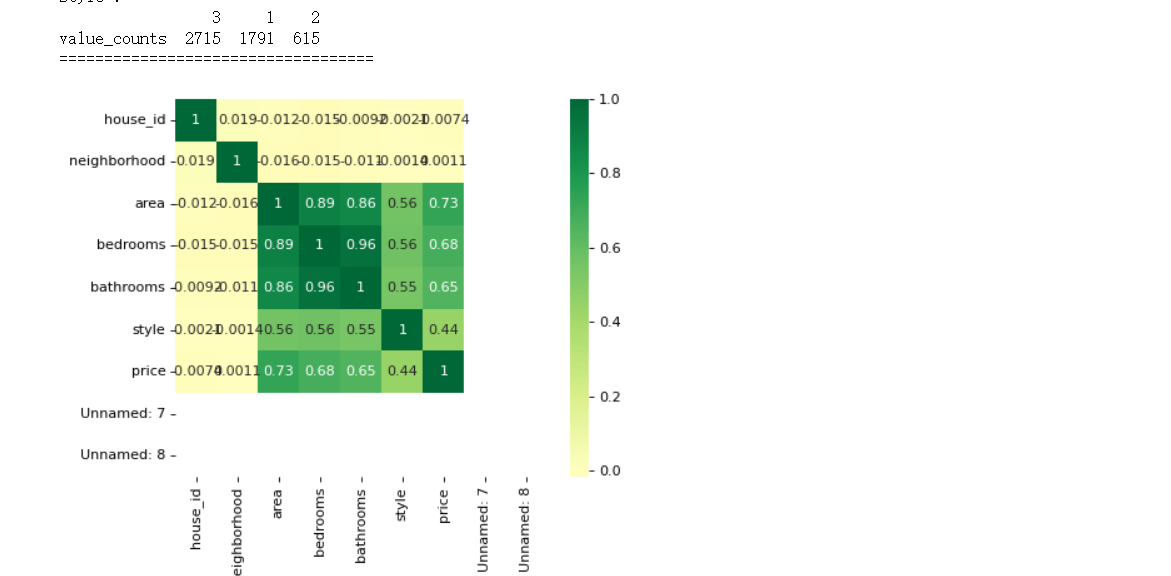2.3、模型拟合

利用回归模型中的方差分析，从线性回归结果中提取方差分析结果
代码：

import statsmodels.api as sm
from statsmodels.formula.api import ols # ols 为建立线性回归模型的统计学库
from statsmodels.stats.anova import anova_lm

随机抽取600条数据样本

df = df.copy().sample(600)

# C 表示告诉 Python 这是分类变量，否则 Python 会当成连续变量使用
## 这里直接使用方差分析对所有分类变量进行检验
## 下面几行代码便是使用统计学库进行方差分析的标准姿势
lm = ols('price ~ C(neighborhood) + C(style)', data=df).fit()
anova_lm(lm)

# Residual 行表示模型不能解释的组内的，其他的是能解释的组间的
# df: 自由度（n-1）- 分类变量中的类别个数减1
# sum_sq: 总平方和（SSM），residual行的 sum_eq: SSE
# mean_sq: msm, residual行的 mean_sq: mse
# F：F 统计量，查看卡方分布表即可
# PR(>F): P 值

# 反复刷新几次，发现都很显著，所以这两个变量也挺值得放入模型中

得到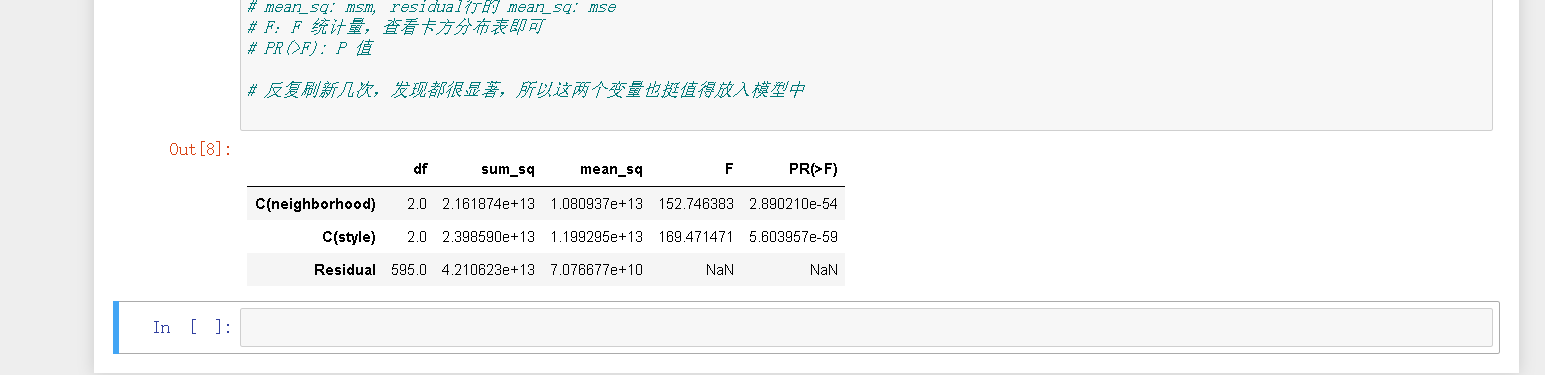建立多元线性回归模型

from statsmodels.formula.api import ols

lm = ols('price ~ area + bedrooms + bathrooms', data=df).fit()
lm.summary()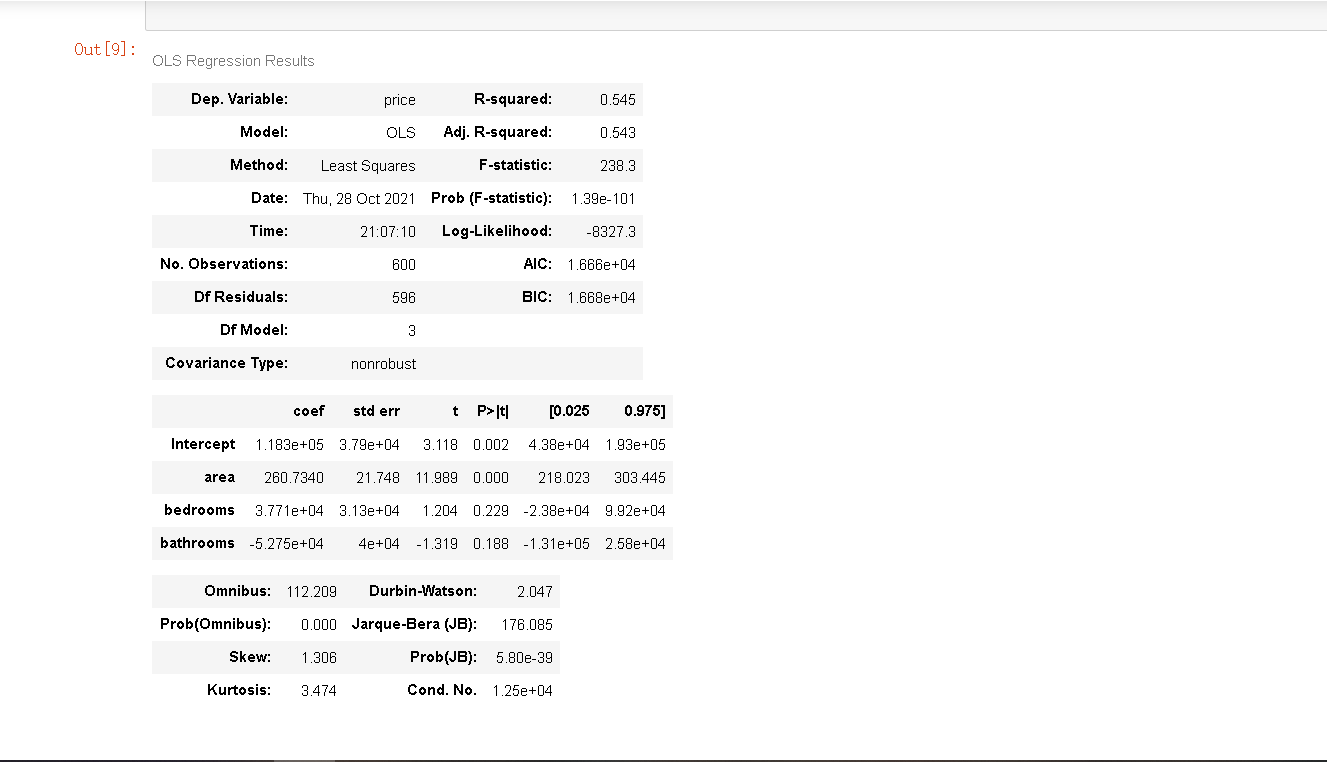二、Excel实现多元线性回归，求解回归方程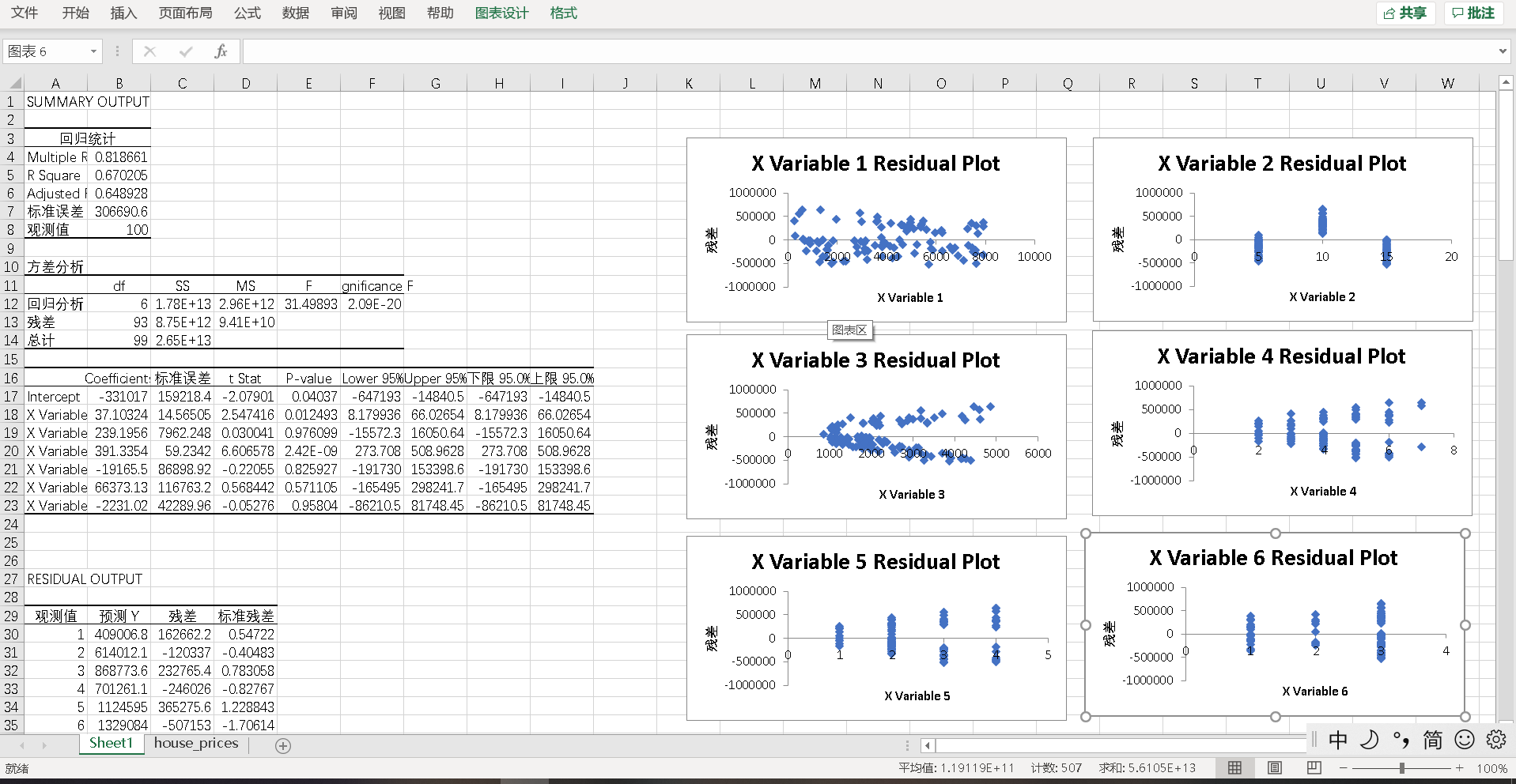2、设因变量房屋售价为y，自变量房屋编号为x1，自变量街区为x2，自变量卧室面积为x3，自变量总面积为x4，自变量浴室面积为x5，自变量房屋风格为x6，在上图的表中，Coefficients为常数项和X Variable的值，据此便可以估算得出回归方程为：y= 37.1024* x1+ 239.1956* x2+391.3354* x3-19165.5x4+66373.13x5-2231.02*x6-331017。但根据Coefficients估算出的回归方程可能存在较大的误差，在第三张子表中更为重要的一列是P-value列，P-value为回归系数t统计量的P值。由表中P-value的值可以发现，自变量房屋总面积的P值小于显著性水平0.05，因此这个自变量与y相关。浴室面积和卧室面积的P值大于显著性水平0.05，说这两个自变量与y相关性较弱，甚至不存在线性相关关系。

三、Sklearn库实现多元线性回归，对结果进行对比分析

3.1、初次线性回归

导入相关包和没有处理过的数据数据

import pandas as pd
import numpy as np
import seaborn as sns
from sklearn import datasets
from sklearn.linear_model import LinearRegression
df.info()#显示列名和数据类型类型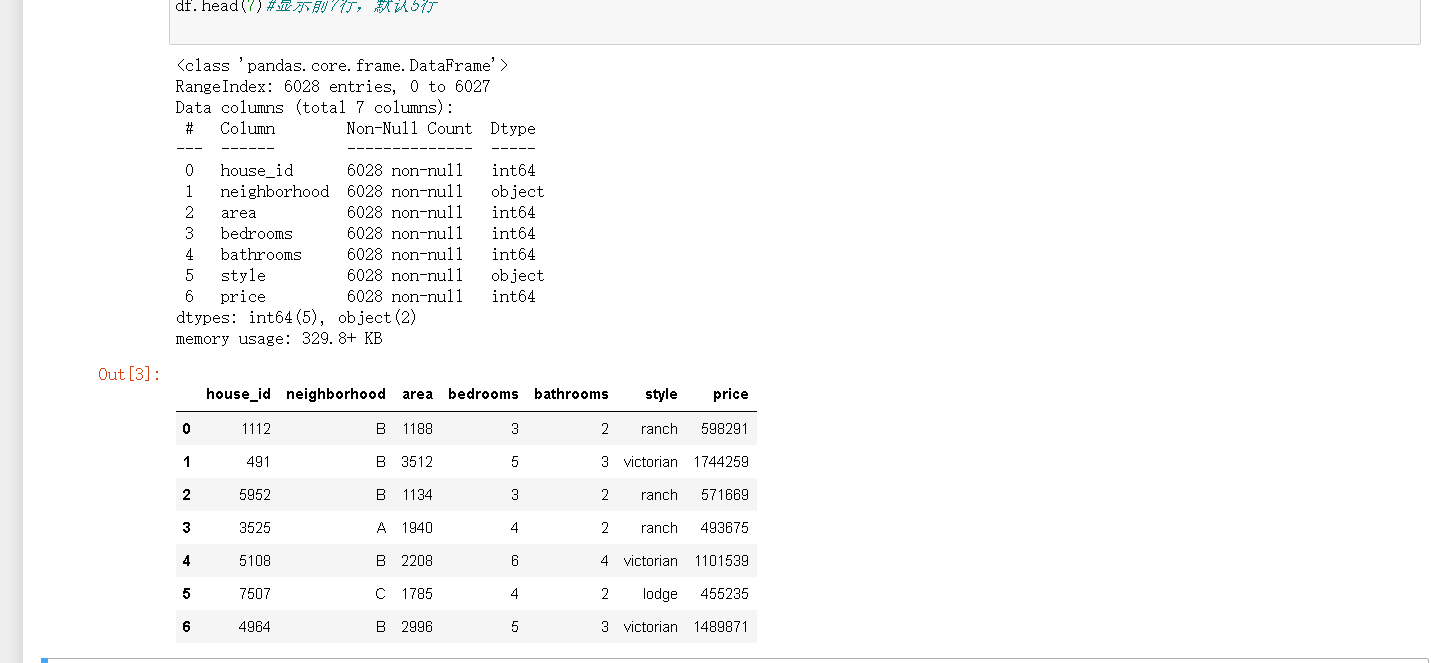实现多元线性回归

# 读取数据
data_x=df[['area','bedrooms','bathrooms']]
data_y=df['price']
# 进行多元线性回归
model=LinearRegression()
l_model=model.fit(data_x,data_y)
print('回归系数')
print(model.coef_)
print('截距')
print(model.intercept_)
print('回归方程: Y=(',model.coef_,')*x1 +(',model.coef_,')*x2 +(',model.coef_,')*x3 +(',model.intercept_,')')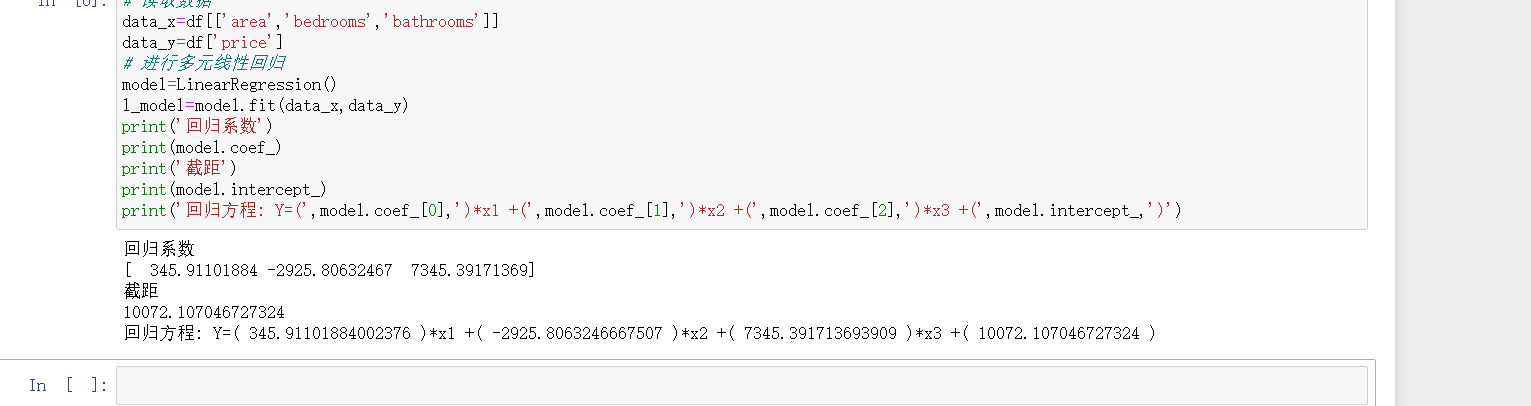3.2、数据处理并再次模拟

进行异常数据处理

# 异常值处理
# ================ 异常值检验函数：iqr & z分数 两种方法 =========================
def outlier_test(data, column, method=None, z=2):
""" 以某列为依据，使用 上下截断点法 检测异常值(索引) """
"""
full_data: 完整数据
column: full_data 中的指定行，格式 'x' 带引号
return 可选; outlier: 异常值数据框
upper: 上截断点;  lower: 下截断点
method：检验异常值的方法（可选, 默认的 None 为上下截断点法），
选 Z 方法时，Z 默认为 2
"""
# ================== 上下截断点法检验异常值 ==============================
if method == None:
print(f'以 {column} 列为依据，使用 上下截断点法(iqr) 检测异常值...')
print('=' * 70)
# 四分位点；这里调用函数会存在异常
column_iqr = np.quantile(data[column], 0.75) - np.quantile(data[column], 0.25)
# 1，3 分位数
(q1, q3) = np.quantile(data[column], 0.25), np.quantile(data[column], 0.75)
# 计算上下截断点
upper, lower = (q3 + 1.5 * column_iqr), (q1 - 1.5 * column_iqr)
# 检测异常值
outlier = data[(data[column] <= lower) | (data[column] >= upper)]
print(f'第一分位数: {q1}, 第三分位数：{q3}, 四分位极差：{column_iqr}')
print(f"上截断点：{upper}, 下截断点：{lower}")
return outlier, upper, lower
# ===================== Z 分数检验异常值 ==========================
if method == 'z':
""" 以某列为依据，传入数据与希望分段的 z 分数点，返回异常值索引与所在数据框 """
"""
params
data: 完整数据
column: 指定的检测列
z: Z分位数, 默认为2，根据 z分数-正态曲线表，可知取左右两端的 2%，
根据您 z 分数的正负设置。也可以任意更改，知道任意顶端百分比的数据集合
"""
print(f'以 {column} 列为依据，使用 Z 分数法，z 分位数取 {z} 来检测异常值...')
print('=' * 70)
# 计算两个 Z 分数的数值点
mean, std = np.mean(data[column]), np.std(data[column])
upper, lower = (mean + z * std), (mean - z * std)
print(f"取 {z} 个 Z分数：大于 {upper} 或小于 {lower} 的即可被视为异常值。")
print('=' * 70)
# 检测异常值
outlier = data[(data[column] <= lower) | (data[column] >= upper)]
return outlier, upper, lower
outlier, upper, lower = outlier_test(data=df, column='price', method='z')
outlier.info(); outlier.sample(5)
# 这里简单的丢弃即可
df.drop(index=outlier.index, inplace=True)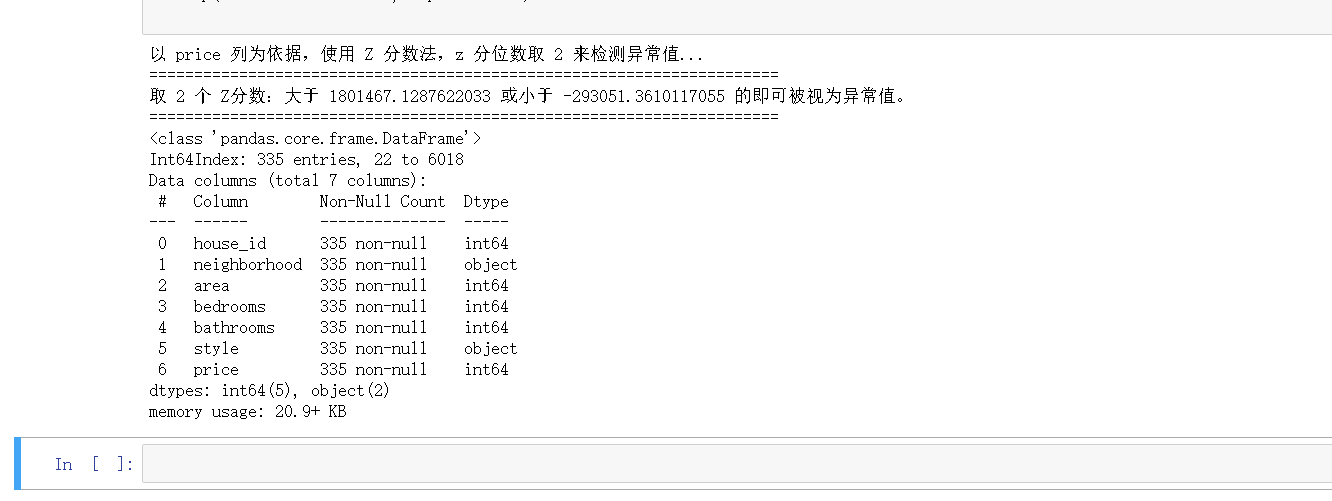再次进行回归模型模拟

# 读取数据
data_x=df[['area','bedrooms','bathrooms']]
data_y=df['price']
# 进行多元线性回归
model=LinearRegression()
l_model=model.fit(data_x,data_y)
print('回归系数')
print(model.coef_)
print('截距')
print(model.intercept_)
print('回归方程: Y=(',model.coef_,')*x1 +(',model.coef_,')*x2 +(',model.coef_,')*x3 +(',model.intercept_,')')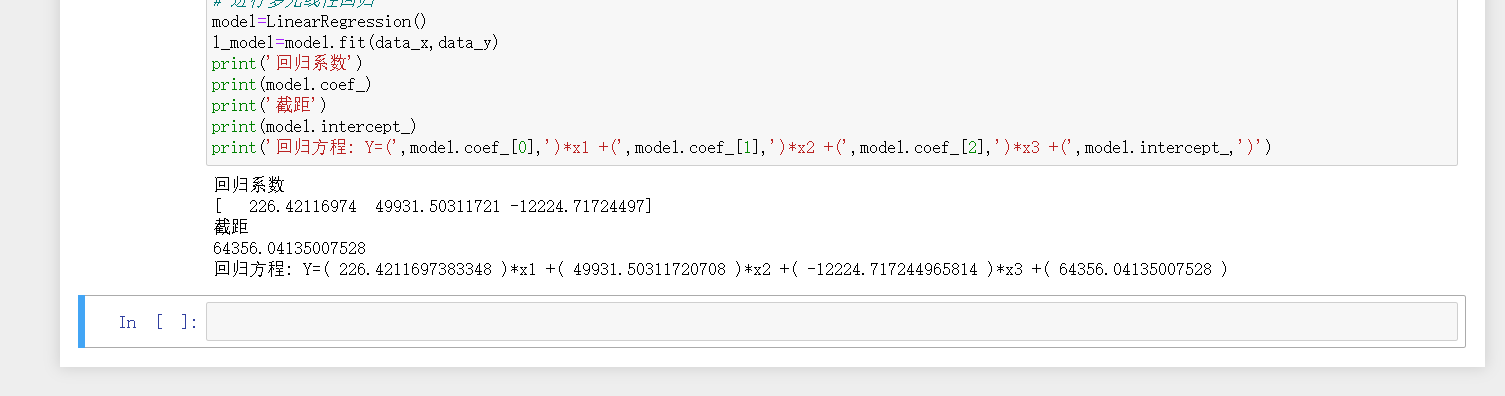参考：回归模型

展开全文线性回归 算法 回归
• house price

2020-10-13 17:47:19

Kaggle房价预测

供个人学习复习用

import pandas as pd
import numpy as np
import matplotlib.pyplot as plt
import seaborn as sns
from sklearn import ensemble, tree, linear_model
from sklearn.model_selection import train_test_split, cross_val_score
from sklearn.metrics import r2_score, mean_squared_error
from sklearn.utils import shuffle

%matplotlib inline
import warnings
warnings.filterwarnings('ignore')

train = train_data.copy()
test = test_data.copy()train.shape,test.shape#check for dupes for Id
idsUnique = len(set(train.Id))#set是集合
idsTotal = train.shape
#这里是集合过滤重复id，只余下唯一值，然后总数减去唯一值查看不重复的数量
idsdupe = idsTotal - idsUnique
print(idsdupe)  #输出是0
#drop id col
train.drop(['Id'],axis=1,inplace=True)

进行可视化

#correlation matrix相关矩阵
corrmat = train.corr()
f,ax = plt.subplots(figsize=(20,9))
sns.heatmap(corrmat,vmax=.8,annot=True)# most correlated features
corrmat = train.corr()
top_corr_features = corrmat.index[abs(corrmat['SalePrice'])>0.5]#corrmat.index取出所有特征名，然后取出与特征SalePrice相关性大于0.5的其他特征
plt.figure(figsize=(10,10))
g = sns.heatmap(train[top_corr_features].corr(),annot=True,cmap='RdYlGn')#再查看这些特征与特征之间的相关性#我们将在下图中看到OverallQual如何影响销售价格。(因为它与销售价格高度相关)
sns.barplot(train.OverallQual,train.SalePrice)#下面可以看到每一个特征与销售价格之间的关联
sns.set()
cols = ['SalePrice', 'OverallQual', 'GrLivArea', 'GarageCars', 'TotalBsmtSF', 'FullBath', 'YearBuilt']
sns.pairplot(train[cols],size=2.5)
plt.show()因为最终目的是要预测销售价格，所以下面可以进行对改变量进行分析

from scipy import stats
from scipy.stats import norm, skew #for some statistics，norm实现正态分布，skew表示概率分布密度曲线相对于平均值不对称程度的特征数，也即偏度
#skew直观来看就是密度函数曲线尾部的相对长度
sns.distplot(train['SalePrice'] , fit=norm);#正态分布曲线拟合图
#通过函数获取拟合参数（Get the fitted parameters used by the function）
#!!!!!!!!!!!!!!!!!!!!!!!!!!!!!!!!!!
(mu, sigma) = norm.fit(train['SalePrice'])#返回mu均值，sigma是方差
print( '\n mu = {:.2f} and sigma = {:.2f}\n'.format(mu, sigma))    #{:,2f}是保留两位小数
plt.legend(['Normal dist. ($\mu=$ {:.2f} and $\sigma=$ {:.2f} )'.format(mu, sigma)],
loc='best')
plt.ylabel('Frequency')
plt.title('SalePrice distribution')

fig = plt.figure()
res = stats.probplot(train['SalePrice'], plot=plt)  #检验样本数据概率分布图（如正态分布（默认））的方法
plt.show()train.SalePrice = np.log1p(train.SalePrice)#对销售价格进行平滑处理（即将数据压缩到一个区间，逆运算是expm1）
y = train.SalePrice#进行加工预处理,查看两个特征之间的散点图
plt.scatter(y=train.SalePrice,x=train.GrLivArea,c='black')
plt.show()train_nas = train.isnull().sum()#计算每个特征的空值总数
train_nas = train_nas[train_nas>0]#筛选出有空值的特征
train_nas.sort_values(ascending = False)#按空值数量进行排序#同理对训练集进行相同的操作
test_nas = test.isnull().sum()
test_nas = test_nas[test_nas>0]
test_nas.sort_values(ascending = False)print("Find most important features relative to target")
corr = train.corr()#得到特征之间的相关性矩阵
corr.sort_values(['SalePrice'],ascending=False,inplace=True)#按照列（特征）SalePrice进行排序
print(corr.SalePrice)#区分数字特征（减去目标）和分类特征,Differentiate numerical features (minus the target) and categorical features
categorical_features = train.select_dtypes(include=['object']).columns#只获取分类特征
categorical_featuresnumerical_features = train.select_dtypes(exclude = ["object"]).columns#获取非分类特征
numerical_features

categorical_features = train.select_dtypes(include = ["object"]).columns
numerical_features = train.select_dtypes(exclude = ["object"]).columns
numerical_features = numerical_features.drop("SalePrice")#非分类特征中删去目标值（销售价格）
print("Numerical features : " + str(len(numerical_features)))
print("Categorical features : " + str(len(categorical_features)))
train_num = train[numerical_features]
train_cat = train[categorical_features]#使用mean（）来填充na值，实际上在进行特征工程时有很多需要探索的地方。
#NOTE: i simply used median() to fill na values, actually there is lot to explore when you do feature engineering. But this notebook aim is to simplify things(no heavy code)

## Handle remaining missing values for numerical features by using median as replacement
#使用中位数来填充处理数值特征缺失的部分
print('NAs for numerical features in train:' + str(train_num.isnull().values.sum()))
train_num = train_num.fillna(train_num.median())
print('Remaining NAs for numerical features in train:'+str(train_num.isnull().values.sum()))from scipy.stats import skew
skewness = train_num.apply(lambda x:skew(x))#遍历每一列，将每一列都调用匿名函数
skewness.sort_values(ascending=False)skewness = skewness[abs(skewness)>0.5]
skewness.index#取dataframe的特征名，---没有复制过来图片

skew_features = train[skewness.index]#从训练集中选出已经挑选出的特征，（它们是非分类型特征且这些特征之间的不对称度大于0.5）
skew_features.columns#we can treat skewness of a feature with the help fof log transformation.so we'll apply the same here.
#借助对数转换来处理特征的偏斜度，因此我们将在此处应用相同的偏度。
skew_features = np.log1p(skew_features)  #将目标矩阵skew_features中的值全部取对数str(train_cat.isnull().values.sum())#查看非分类特征中有无空值---0

下面开始进行模型

import pandas as pd
import numpy as np
from sklearn.model_selection import cross_val_score, train_test_split
from sklearn.preprocessing import StandardScaler
from sklearn.linear_model import LinearRegression, RidgeCV, LassoCV, ElasticNetCV
from sklearn.metrics import mean_squared_error, make_scorer #metrics 是指标，make_scorer从性能指标或损失函数中创建一个计分标准
import matplotlib.pyplot as plt
import seaborn as sns

train = pd.concat([train_cat,train_num],axis=1)#将预处理的训练集合并（原本分为了分类集和非分类集，用来预处理）
X_train,X_test,y_train,y_test = train_test_split(train,y,test_size = 0.3,random_state= 0)

#用交叉验证集分布检测训练集和测试集
n_folds = 5
from sklearn.metrics import make_scorer
from sklearn.model_selection import KFold#K折交叉验证
scorer = make_scorer(mean_squared_error,greater_is_better = False)
def rmse_CV_train(model):
kf = KFold(n_folds,shuffle=True,random_state=42).get_n_splits(train.values)#在K折交叉验证中将训练集再次划分
rmse = np.sqrt(-cross_val_score(model,X_train,y_train,scoring ="neg_mean_squared_error",cv=kf))
return (rmse)
def rmse_CV_test(model):
kf = KFold(n_folds,shuffle=True,random_state=42).get_n_splits(train.values)
rmse = np.sqrt(-cross_val_score(model,X_test,y_test,scoring ="neg_mean_squared_error",cv=kf))
return (rmse)

#Linear model without Regularization
lr = LinearRegression()
lr.fit(X_train,y_train)
test_pre = lr.predict(X_test)
train_pre = lr.predict(X_train)
print('rmse on train',rmse_CV_train(lr).mean())
print('rmse on train',rmse_CV_test(lr).mean())#plot between predicted values and residuals
plt.scatter(train_pre, train_pre - y_train, c = "blue",  label = "Training data")#残差即预测值与真实值之间的差异
plt.scatter(test_pre,test_pre - y_test, c = "black",  label = "Validation data")
plt.title("Linear regression")
plt.xlabel("Predicted values")
plt.ylabel("Residuals")
plt.legend(loc = "upper left")
plt.hlines(y = 0, xmin = 10.5, xmax = 13.5, color = "red")
plt.show()# Plot predictions - Real values绘画真实值和预测值散点图
plt.scatter(train_pre, y_train, c = "blue",  label = "Training data")
plt.scatter(test_pre, y_test, c = "black",  label = "Validation data")
plt.title("Linear regression")
plt.xlabel("Predicted values")
plt.ylabel("Real values")
plt.legend(loc = "upper left")
plt.plot([10.5, 13.5], [10.5, 13.5], c = "red")
plt.show()正则化是处理共线性，从数据中滤除噪声并最终防止过度拟合的非常有用的方法。
正则化背后的概念是引入附加信息（偏差）以惩罚极端参数权重。

Regularization is a very useful method to handle collinearity, filter out noise from data, and eventually prevent overfitting.
The concept behind regularization is to introduce additional information (bias) to penalize extreme parameter weights.#RidgeCV内置交叉验证的岭回归，默认情况下，它执行通用的交叉验证，这是一种有效的留一交叉验证的形式。alpha是正则化的力度
#Ridge:固定阿尔法，求出最佳w，阿尔法与w的范数成反比，
#RidgeCV:多个阿尔法，得出多个对应最佳的w,然后得到最佳的w及对应的阿尔法
ridge = RidgeCV(alphas = [0.01, 0.03, 0.06, 0.1, 0.3, 0.6, 1, 3, 6, 10, 30, 60])

ridge.fit(X_train,y_train)
alpha = ridge.alpha_#一轮下来得到最好的alpha
print('best alpha',alpha)

print("Try again for more precision with alphas centered around " + str(alpha))
ridge = RidgeCV(alphas = [alpha * .6, alpha * .65, alpha * .7, alpha * .75, alpha * .8, alpha * .85,
alpha * .9, alpha * .95, alpha, alpha * 1.05, alpha * 1.1, alpha * 1.15,
alpha * 1.25, alpha * 1.3, alpha * 1.35, alpha * 1.4],cv = 5)
ridge.fit(X_train, y_train)
alpha = ridge.alpha_
print("Best alpha :", alpha)
print("Ridge RMSE on Training set :", rmse_CV_train(ridge).mean())#K折交叉验证结果的均值
print("Ridge RMSE on Test set :", rmse_CV_test(ridge).mean())
y_train_rdg = ridge.predict(X_train)#岭回归的返回分数
y_test_rdg = ridge.predict(X_test)print("Kcv RMSE on Training set :", y_train_rdg.mean())#K折交叉验证结果的均值
print("Kcv RMSE on Test set :", y_test_rdg.mean())coef = pd.Series(ridge.coef_, index = X_train.columns)

print("Ridge picked " + str(sum(coef != 0)) + " variables and eliminated the other " +  str(sum(coef == 0)) + " variables")# Plot residuals
plt.scatter(y_train_rdg, y_train_rdg - y_train, c = "blue",  label = "Training data")
plt.scatter(y_test_rdg, y_test_rdg - y_test, c = "black", marker = "v", label = "Validation data")
plt.title("Linear regression with Ridge regularization")
plt.xlabel("Predicted values")
plt.ylabel("Residuals")
plt.legend(loc = "upper left")
plt.hlines(y = 0, xmin = 10.5, xmax = 13.5, color = "red")
plt.show()# Plot predictions - Real values
plt.scatter(y_train_rdg, y_train, c = "blue",  label = "Training data")
plt.scatter(y_test_rdg, y_test, c = "black",  label = "Validation data")
plt.title("Linear regression with Ridge regularization")
plt.xlabel("Predicted values")
plt.ylabel("Real values")
plt.legend(loc = "upper left")
plt.plot([10.5, 13.5], [10.5, 13.5], c = "red")
plt.show()展开全文机器学习 深度学习
• In this exercise, you'll work on the "Happy House" problem, which we'll explain below. Let's load the required packages and solve the problem of the Happy House! 目录 1 - The Happy House 2 ...

本文节选自吴恩达老师《深度学习专项课程》编程作业，在此表示感谢。

Welcome to the first assignment of week 2. In this assignment, you will:

1. Learn to use Keras, a high-level neural networks API (programming framework), written in Python and capable of running on top of several lower-level frameworks including TensorFlow and CNTK.
2. See how you can in a couple of hours build a deep learning algorithm.

Why are we using Keras? Keras was developed to enable deep learning engineers to build and experiment with different models very quickly. Just as TensorFlow is a higher-level framework than Python, Keras is an even higher-level framework and provides additional abstractions. Being able to go from idea to result with the least possible delay is key to finding good models. However, Keras is more restrictive than the lower-level frameworks, so there are some very complex models that you can implement in TensorFlow but not (without more difficulty) in Keras. That being said, Keras will work fine for many common models.

In this exercise, you'll work on the "Happy House" problem, which we'll explain below. Let's load the required packages and solve the problem of the Happy House!

目录

1 - The Happy House

2 - Building a model in Keras

3 - Conclusion

4 - Other useful functions in Keras

import numpy as np
#import tensorflow as tf
from keras import layers
from keras.layers import Input, Dense, Activation, ZeroPadding2D, BatchNormalization, Flatten, Conv2D
from keras.layers import AveragePooling2D, MaxPooling2D, Dropout, GlobalMaxPooling2D, GlobalAveragePooling2D
from keras.models import Model
from keras.preprocessing import image
from keras.utils import layer_utils
from keras.utils.data_utils import get_file
from keras.applications.imagenet_utils import preprocess_input
import pydot
from IPython.display import SVG
from keras.utils.vis_utils import model_to_dot
from keras.utils import plot_model
from kt_utils import *

import keras.backend as K
K.set_image_data_format('channels_last')
import matplotlib.pyplot as plt
from matplotlib.pyplot import imshow

%matplotlib inline

Note: As you can see, we've imported a lot of functions from Keras. You can use them easily just by calling them directly in the notebook. Ex: X = Input(...) or X = ZeroPadding2D(...).

1 - The Happy House

For your next vacation, you decided to spend a week with five of your friends from school. It is a very convenient house with many things to do nearby. But the most important benefit is that everybody has commited to be happy when they are in the house. So anyone wanting to enter the house must prove their current state of happiness.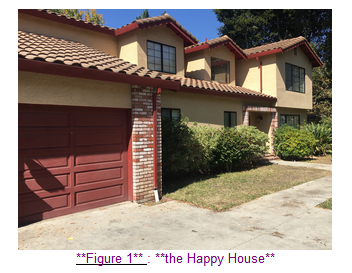As a deep learning expert, to make sure the "Happy" rule is strictly applied, you are going to build an algorithm which that uses pictures from the front door camera to check if the person is happy or not. The door should open only if the person is happy.

You have gathered pictures of your friends and yourself, taken by the front-door camera. The dataset is labbeled.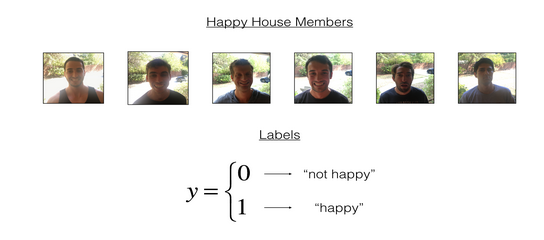X_train_orig, Y_train_orig, X_test_orig, Y_test_orig, classes = load_dataset()

# Normalize image vectors
X_train = X_train_orig/255.
X_test = X_test_orig/255.

# Reshape
Y_train = Y_train_orig.T
Y_test = Y_test_orig.T

print ("number of training examples = " + str(X_train.shape))
print ("number of test examples = " + str(X_test.shape))
print ("X_train shape: " + str(X_train.shape))
print ("Y_train shape: " + str(Y_train.shape))
print ("X_test shape: " + str(X_test.shape))
print ("Y_test shape: " + str(Y_test.shape))

2 - Building a model in Keras

Keras is very good for rapid prototyping. In just a short time you will be able to build a model that achieves outstanding results.

Here is an example of a model in Keras:

def model(input_shape):
# Define the input placeholder as a tensor with shape input_shape. Think of this as your input image!
X_input = Input(input_shape)

# CONV -> BN -> RELU Block applied to X
X = Conv2D(32, (7, 7), strides = (1, 1), name = 'conv0')(X)
X = BatchNormalization(axis = 3, name = 'bn0')(X)
X = Activation('relu')(X)

# MAXPOOL
X = MaxPooling2D((2, 2), name='max_pool')(X)

# FLATTEN X (means convert it to a vector) + FULLYCONNECTED
X = Flatten()(X)
X = Dense(1, activation='sigmoid', name='fc')(X)

# Create model. This creates your Keras model instance, you'll use this instance to train/test the model.
model = Model(inputs = X_input, outputs = X, name='HappyModel')

return model

Note that Keras uses a different convention with variable names than we've previously used with numpy and TensorFlow. In particular, rather than creating and assigning a new variable on each step of forward propagation such as X, Z1, A1, Z2, A2, etc. for the computations for the different layers, in Keras code each line above just reassigns X to a new value using X = .... In other words, during each step of forward propagation, we are just writing the latest value in the commputation into the same variable X. The only exception was X_input, which we kept separate and did not overwrite, since we needed it at the end to create the Keras model instance (model = Model(inputs = X_input, ...) above).

Exercise: Implement a HappyModel(). This assignment is more open-ended than most. We suggest that you start by implementing a model using the architecture we suggest, and run through the rest of this assignment using that as your initial model. But after that, come back and take initiative to try out other model architectures. For example, you might take inspiration from the model above, but then vary the network architecture and hyperparameters however you wish. You can also use other functions such as AveragePooling2D(), GlobalMaxPooling2D(), Dropout().

Note: You have to be careful with your data's shapes. Use what you've learned in the videos to make sure your convolutional, pooling and fully-connected layers are adapted to the volumes you're applying it to.

def HappyModel(input_shape):
"""
Implementation of the HappyModel.

Arguments:
input_shape -- shape of the images of the dataset

Returns:
model -- a Model() instance in Keras
"""

# Feel free to use the suggested outline in the text above to get started, and run through the whole
# exercise (including the later portions of this notebook) once. The come back also try out other
# network architectures as well.

X_input = Input(input_shape)

x = Conv2D(8, (3,3), strides=(1,1))(X)
X = BatchNormalization(axis=3)(X)
X = Activation('relu')(X)

X = Conv2D(16, kernel_size=(3,3), strides=(1,1))(X)
X = BatchNormalization(axis=3)(X)
X = Activation('relu')(X)

X = Conv2D(32, kernel_size=(3,3), strides=(1,1))(X)
X = BatchNormalization(axis=3)(X)
X = Activation('relu')(X)

# FC
X = Flatten()(X)
Y = Dense(1, activation='sigmoid')(X)

model = Model(inputs = X_input, outputs = Y, name='HappyModel')

return model

You have now built a function to describe your model. To train and test this model, there are four steps in Keras:

1. Create the model by calling the function above
2. Compile the model by calling model.compile(optimizer = "...", loss = "...", metrics = ["accuracy"])
3. Train the model on train data by calling model.fit(x = ..., y = ..., epochs = ..., batch_size = ...)
4. Test the model on test data by calling model.evaluate(x = ..., y = ...)

If you want to know more about model.compile(), model.fit(), model.evaluate() and their arguments, refer to the official Keras documentation.

Exercise: Implement step 1, i.e. create the model.

happyModel = HappyModel((64,64,3))

Exercise: Implement step 2, i.e. compile the model to configure the learning process. Choose the 3 arguments of compile() wisely. Hint: the Happy Challenge is a binary classification problem.

import keras

happyModel.compile(optimizer=keras.optimizers.Adam(lr=0.001, beta_1=0.9, beta_2=0.999, epsilon=1e-08, decay=0.0), loss='binary_crossentropy', metrics=['accuracy'])

Exercise: Implement step 3, i.e. train the model. Choose the number of epochs and the batch size.

happyModel.fit(x=X_train, y=Y_train, batch_size=16, epochs=20)

preds = happyModel.evaluate(x=X_test, y=Y_test)

print()
print ("Loss = " + str(preds))
print ("Test Accuracy = " + str(preds))

If your happyModel() function worked, you should have observed much better than random-guessing (50%) accuracy on the train and test sets. To pass this assignment, you have to get at least 75% accuracy.

To give you a point of comparison, our model gets around 95% test accuracy in 40 epochs (and 99% train accuracy) with a mini batch size of 16 and "adam" optimizer. But our model gets decent accuracy after just 2-5 epochs, so if you're comparing different models you can also train a variety of models on just a few epochs and see how they compare.

If you have not yet achieved 75% accuracy, here're some things you can play around with to try to achieve it:

• Try using blocks of CONV->BATCHNORM->RELU such as:
X = Conv2D(32, (3, 3), strides = (1, 1), name = 'conv0')(X)
X = BatchNormalization(axis = 3, name = 'bn0')(X)
X = Activation('relu')(X)
until your height and width dimensions are quite low and your number of channels quite large (≈32 for example). You are encoding useful information in a volume with a lot of channels. You can then flatten the volume and use a fully-connected layer.
• You can use MAXPOOL after such blocks. It will help you lower the dimension in height and width.
• If the model is struggling to run and you get memory issues, lower your batch_size (12 is usually a good compromise)
• Run on more epochs, until you see the train accuracy plateauing.

Even if you have achieved 75% accuracy, please feel free to keep playing with your model to try to get even better results.

Note: If you perform hyperparameter tuning on your model, the test set actually becomes a dev set, and your model might end up overfitting to the test (dev) set. But just for the purpose of this assignment, we won't worry about that here.

3 - Conclusion

Congratulations, you have solved the Happy House challenge!

Now, you just need to link this model to the front-door camera of your house. We unfortunately won't go into the details of how to do that here.

**What we would like you to remember from this assignment:** - Keras is a tool we recommend for rapid prototyping. It allows you to quickly try out different model architectures. Are there any applications of deep learning to your daily life that you'd like to implement using Keras? - Remember how to code a model in Keras and the four steps leading to the evaluation of your model on the test set. Create->Compile->Fit/Train->Evaluate/Test.

4 - Other useful functions in Keras

wo other basic features of Keras that you'll find useful are:

• model.summary(): prints the details of your layers in a table with the sizes of its inputs/outputs
• plot_model(): plots your graph in a nice layout. You can even save it as ".png" using SVG() if you'd like to share it on social media ;). It is saved in "File" then "Open..." in the upper bar of the notebook.

Run the following code.

happyModel.summary()

plot_model(happyModel, to_file='HappyModel.png')
SVG(model_to_dot(happyModel).create(prog='dot', format='svg'))

展开全文• 综述house of spirit是一种常用的堆溢出技术，而在如今的malloc实现中依然没有对这种方法进行保护，所以在目前还是一种有效的堆溢出技术。下面我们先从这种方法的来源之本讲起，即2005 Malloc Maleficarumcsdn原文 ...

综述

house of spirit是一种常用的堆溢出技术，而在如今的malloc实现中依然没有对这种方法进行保护，所以在目前还是一种有效的堆溢出技术。下面我们先从这种方法的来源之本讲起，即2005 Malloc Maleficarum

原文

The House of Spirit

The House of Spirit is primarily interesting because of the nature
of the circumstances leading to its application. It is the only
House in the Malloc Maleficarum that can be used to leverage both a
heap and stack overflow. This is because the first step is not to
control the header information of a chunk, but to control a pointer
that is passed to free(). Whether this pointer is on the heap or
not is largely irrelevant.

The general idea involves overwriting a pointer that was previously
returned by a call to malloc(), and that is subsequently passed to
fastbin. A further call to malloc() can result in this arbitrary
address being used as a chunk of memory by the application. If the
designer can control the applications use of the fake chunk, then
it is possible to overwrite execution control data.

Assume that the designer has overflowed a pointer that is being
passed to free(). The first problem that must be considered is
exactly what the pointer should be overflowed with. Keep in mind
that the ultimate goal of the House of Spirit is to allow the
designer to overwrite some sort of execution control data by
returning an arbitrary chunk to the application. Exactly what
"execution control data" is doesn't particularly matter so long as
overflowing it can result in execution being passed to a designer
controlled memory location. The two most common examples that are
suitable for use with the House of Spirit are function pointers and
pending saved return addresses, which will herein be referred to as
the "target".

In order to successfully apply the House of Spirit it is necessary
to have a designer controlled word value at a lower address than
the target. This word will correspond to the size field of the
chunk header for the fakechunk passed to free(). This means that
the overflowed pointer must be set to the address of the designer
controlled word plus 4. Furthermore, the size of the fakechunk must
be must be located no more than 64 bytes away from the target. This
is because the default maximum data size for a fastbin entry is 64,
and at least the last 4 bytes of data are required to overwrite the
target.

There is one more requirement for the layout of the fakechunk data
which will be described shortly. For the moment, assume that all of
the above conditions have been met, and that a call to free() is
made on the suitable fakechunk. A call to free() is handled by a
wrapper function called public_fREe():

void
public_fREe(Void_t* mem)
{
mstate ar_ptr;
mchunkptr p;          /* chunk corresponding to mem */
...
p = mem2chunk(mem);
if (chunk_is_mmapped(p))
{
munmap_chunk(p);
return;
}
...
ar_ptr = arena_for_chunk(p);
...
_int_free(ar_ptr, mem);

In this situation mem is the value that was originally overflowed
to point to a fakechunk. This is converted to the "corresponding
chunk" of the fakechunk's data, and passed to arena_for_chunk() in
order to find the corresponding arena. In order to avoid special
treatment as an mmap() chunk, and also to get a sensible arena, the
size field of the fakechunk header must have the IS_MMAPPED and
NON_MAIN_ARENA bits cleared. To do this, the designer can simply
ensure that the fake size is a multiple of 8. This would mean the
internal function _int_free() is reached:

void_int_free(mstate av, Void_t* mem){
mchunkptr       p;           /* chunk corresponding to mem */
INTERNAL_SIZE_T size;        /* its size */
mfastbinptr*    fb;          /* associated fastbin */
...
p = mem2chunk(mem);
size = chunksize(p);
...
if ((unsigned long)(size) <= (unsigned long)(av->max_fast))
{
if (chunk_at_offset (p, size)->size <= 2 * SIZE_SZ
|| __builtin_expect (chunksize (chunk_at_offset (p, size))
>= av->system_mem, 0))
{
errstr = "free(): invalid next size (fast)";
goto errout;
}
...
fb = &(av->fastbins[fastbin_index(size)]);
...
p->fd = *fb;
*fb = p;
}

This is all of the code in free() that concerns the House of
Spirit. The designer controlled value of mem is again converted to
a chunk and the fake size value is extracted. Since size is
designer controlled, the fastbin code can be triggered simply by
ensuring that it is less than av->max_fast, which has a default of
64 + 8. The final point of consideration in the layout of the
fakechunk is the nextsize integrity tests.

Since the size of the fakechunk has to be large enough to encompass
the target, the size of the nextchunk must be at an address higher
than the target. The nextsize integrity tests must be handled for
the fakechunk to be put in a fastbin, which means that there must
be yet another designer controlled value at an address higher than
the target.

The exact location of the designer controlled values directly
depend on the size of the allocation request that will subsequently
be used by the designer to overwrite the target. That is, if an
allocation request of N bytes is made (such that N <= 64), then the
designer's lower value must be within N bytes of the target and
must be equal to (N + 8). This is to ensure that the fakechunk is
put in the right fastbin for the subsequent allocation request.
Furthermore, the designer's upper value must be at (N + 8) bytes
above the lower value to ensure that the nextsize integrity tests
are passed.

If such a memory layout can be achieved, then the address of this
"structure" will be placed in a fastbin. The code for the
subsequent malloc() request that uses this arbitrary fastbin entry
is simple and need not be reproduced here. As far as _int_malloc()
is concerned the fake chunk that it is preparing to return to the
application is perfectly valid. Once this has occurred it is simply
up to the designer to manipulate the application in to overwriting
the target.

翻译

house of spirit因为其应用情况受到广泛关注，他是这篇文章中提到方法里，唯一一种同时可以利用堆和栈溢出的方法。这是因为他第一步不是去控制一个chunk的头信息，而是去控制一个传给free函数的指针，至于这个指针是不是在堆上并没有太大的关系。

他的中心思想主要是重写一个之前由malloc分配然后被放进free里的一个指针，这就会导致一个任意地址被链接进fastbin。之后的某个malloc调用可以导致这个任意地址被分配作为一个chunk，如果攻击者可以控制这个fake chunk的应用，那么就有机会可以重写关于执行控制的数据。

假设攻击者溢出了一个被放入free调用的指针，需要考虑的第一个问题是用什么来溢出后填充这个指针。需记住的是house of spirit的最终目的是允许攻击者通过返回给这个应用一个任意位置的chunk来重写某些执行控制数据，至于执行控制数据具体是什么并不是太重要只要溢出它能够导致攻击者想要的执行内容被传送到攻击者控制的内存地址。两个最为常见最为适合用house of spirit的例子的指针是函数指针和存储的返回地址，
这里我们把他们称作“目标”。

为了成功应用house of spirit，攻击者必须要求能够控制低于目标的地址的一个字值(word value)，这个字(word)将会和被放进free的fake chunk的头的size域对应。这意味着被溢出的指针将会被设置为攻击者控制的字的地址再加上4，以及fake chunk必须离目标不到64字节。这是因为fastbin的默认块大小是64，而至少我们需要最后4个字节来重写目标。

另外，对于fake chunk的数据分布还有一个要求，我们马上将会讲到。现在我们就先假设之前提到的所有要求都已经被满足了，然后一个对free的调用将会在合适的fake chunk上应用。一个对free的调用将会被一个包装函数，名为public_fREe处理:

void
public_fRE(Void_t* mem)
{
mstate ar_ptr;
mchunkptr p; // mem相应的chunk
...
p = mem2chunk(mem);
if (chunk_is_mmapped(p))
{
munmap_chunk(p);
return;
}
...
ar_ptr = arena_for_chunk(p);
...
_int_free(ar_ptr, mem);
}

在这种情况下，mem是之前已经被溢出并使得指向fake chunk的一个值，然后被转换为fake chunk相应的chunk指针，然后被传仅arena_for_chunk来找到相应的arena，为了避免对于mmap chunk的特殊处理，以及为了得到一个有用的arena，fake chunk头的size域的IS_MMAPPED和NON_MAIN_ARENA位必须为0. 为了做到这个，攻击者只需要确认fake 的size是8的倍数就可以了。这样的话，_int_free函数就会被调用了:

void _int_free(mstate av, Void_t* mem)
{
mchunkptr p; // mem相应的chunk
INTERNAL_SIZE_T size; //size，大小
mfastbinptr* fb; //联系的fast bin
...
p = mem2chunk(mem);
size = chunksize(p);
...
if ((unsigned long)(size) <= (unsigned long)(av->max_fast))
{
if (chunk_at_offset(p, size)->size <= 2 * SIZE_SZ
|| __builtin_expect(chunksize(chunk_at_offset(p, size))
>= av->system_mem, 0))
{
errstr = "free(): invalid next size (fast)";
goto errout;
}
...
fb = &(av->fastbins[fastbin_index(size)]);
...
p->fd = *fb;
*fb = p;
}
}

这里是free对于使用house of spirit所需要了解的全部代码了。攻击者控制的mem值再次被转换为chunk指针，然后fake的size值被提取出来。因为size已经是攻击者控制的了，只需要保证这个值小于av->max_fast，fastbin的代码就会被执行了，这里，av->max_fast的默认值为64+8。最后fake chunk的布局需要考虑的是如何通过nextsize正确性的检测。

因为fake chunk的大小必须要足够大才能包裹住目标，所以nextchunk的size的地址必须高于目标。为了能够使得fake chunk被放进fastbin，nextsize一正确性检验必须被处理一下，这就意味着必须有另外一个攻击者控制的值在高于目标的地址出现。

攻击者控制的值的具体位置依赖于将被用来重写目标的分配请求的大小，这就是说，如果一个分配请求了N个字节(N <= 64)，那么这个攻击者可以控制的低于这个目标地址的值必须在离目标的N 字节以内，并且必须等于N + 8。这是为了保证fake chunk被放在了之后分配请求所需要的正确的fastbin里。另外，攻击者能控制的另外一个，高于目标地址的值必须比低于的那个值的地址高出(N + 8)字节来保证nextsize的正确性检测可以通过。

如果满足了这样一个内存布局，那么这个结构的地址将会被放进fastbin里。其后对于这个已经被控制的fastbin块的malloc请求的代码非常简单，这里就不再给出了。只要_int_malloc被调用，那么这个准备被返回的fake chunk就是有效的。只要这种情况发生了，那么操纵应用来重写目标就非常简单了。

展开全文malloc 技术 linux
• rows',1000) # 显示的最大行数 # 数据读取 os.getcwd() os.chdir('C:/Users/Anqi/00 Mechine learning/Kaggle 2_House Price Prediction/House_Data') train = pd.read_csv('train.csv') test = pd.read_csv('test....机器学习
• Keras tutorial - the Happy House¶Welcome to the first assignment of week 2. In this assignment, you will:Learn to use Keras, a high-level neural networks API (programming framework), written in ...吴恩达深度学习 深度学习作业
• Keras tutorial - the Happy House Welcome to the first assignment of week 2. In this assignment, you will: Learn to use Keras, a high-level neural networks API (programming framework), written in ...卷积神经网络
• corrgram(train, order = TRUE, lower.panel= panel.shade, upper.panel = panel.pie, main = "correlogram of all predictors") We can find that average_room_number & lower_class_ratio have a high ...
• 可为Tier1提供涵盖以下内容的测试认证服务： 车载以太网测试认证，包括： ○ 100Base-T1和1000Base-T1 TC8一致性测试，可提供Upper Tester集成服务 ○ Avnu-AVB和TSN一致性测试 ○ AUTOSAR一致性测试 ○ OEM定制测试...测试工程师 autosar
• Provisioning an iOS app for in-house distributione is damn complicated. As my efforts to do so were eventually successful, I decided to prepare this comprehensive tutorial documenting my best practiceipa app ios
• Keras - Tutorial - Happy House v2

千次阅读 2017-11-23 22:00:24
Keras tutorial - the Happy House Welcome to the first assignment of week 2. In this assignment, you will: 1. Learn to use Keras, a high-level neural networks API (programming framework), written ...
• 来自吴恩达深度学习系列视频 卷积神经网络 第三周作业 Keras±+Tutorial±+Happy+House+v1，它是一个Keras的小教程。中文翻译参照：【中文】【吴恩达课后编程作业】Course 4 - 卷积神经网络 - 第二周作业 - Keras...Keras
• How to Build a House

千次阅读 2015-04-10 14:06:51
How to Build a House Seven Parts:Finding a LocationDesigning Your HomeGetting the Necessary PermitsBreaking GroundBuilding the Walls and RoofStarting on the InteriorInstalling the Essentials
• Keras tutorial - the Happy House Welcome to the first assignment of week 2. In this assignment, you will: 1. Learn to use Keras, a high-level neural networks API (programming framework), written ...api 框架 keras 深度学习 coursera
• there is a magical city in the east of china ...however, since the house market is so rich when it is increasing their corresponding profit the corresponding real economics are so poor that t
• Keras tutorial - the Happy House Welcome to the first assignment of week 2. In this assignment, you will: Learn to use Keras, a high-level neural networks API (programming framework), written in Pyt...
• php-cs-fixerOne of my favorite cable television networks has a show called "Flip that House." The concept is as simple as the work is hard. You buy a house that needs work, do the work, and sell it f...大数据 编程语言 区块链 python 人工智能
• 原标题：我们的大屋顶|阳光方舟2.0--C-HOUSE正式封顶屋顶是一所房子最重要的部分，The roof is the most important part of a house.是穹顶，是栖居的庇护。It's the dome. It's the shelter.在这里，人们能够仰望...C语言封顶
• 1Keras tutorial - the Happy House Welcome to the first assignment of week 2. In this assignment, you will: Learn to use Keras, a high-level neural networks API (programming framework), written ...Keras
• 以下来自Coursera深度学习系列...Keras tutorial - the Happy House Welcome to the first assignment of week 2. In this assignment, you will: 1. Learn to use Keras, a high-level neural networks API (p...深度学习 神经网络
• In this exercise, you’ll work on the “Happy House” problem, which we’ll explain below. Let’s load the required packages and solve the problem of the Happy House! import numpy as np from ...class 深度学习 吴恩达
• upper = 0 lower = 0 if len (data) 10 : return False else : for i in range ( len (data)): if data[i].isdigit(): digit += 1 elif data[i].islower(): lower += 1 elif data...
• Predicting house prices is a good example: the different target prices form a continuous space. Vector regression—A task where the target is a set of continuous values: for example, a continuous ...
• and one digit, one upper case and one lower case letter. """ if len(data) return False if not DIGIT_RE.search(data): return False if not UPPER_CASE_RE.search(data): return False if not ......